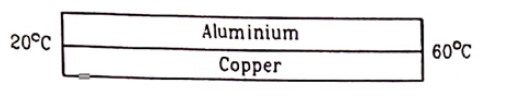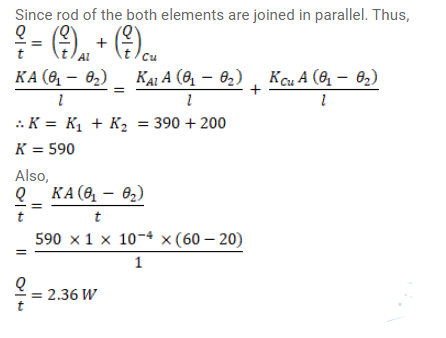# An aluminium rod and a copper rod of equal length`
Question:

An aluminium rod and a copper rod of equal length $1.0 \mathrm{~m}$ and cross - sectional area $1 \mathrm{~cm}^{2}$ are welded together as shown in figure. One end is kept at a temperature of $20^{\circ} \mathrm{C}$ and the other at $60^{\circ} \mathrm{C}$. Calculate the amount of heat taken out per second from the hot end. Thermal conductivity of aluminium $=200 \mathrm{~W} / \mathrm{m}$ ${ }^{\circ} \mathrm{C}$ and of copper $=390 \mathrm{~W} / \mathrm{m}-{ }^{\circ} \mathrm{C}$.Solution: